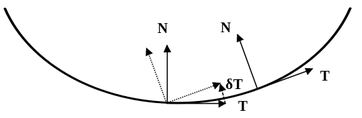# Curvature of a parametric curveLet $$s: \mathbb R \rightarrow \mathbb R^3$$ be a vector valued function representing a parametric curve $$s(t)$$ with $$t \in \mathbb R$$ the curve's parameter; the curvature of $$s$$ is given by $$\kappa: \mathbb R \rightarrow \mathbb R$$ as follows:

$\kappa(t) = \frac{ \| s'(t) \times s''(t) \| }{ \| s'(t) \|^3 }$

Where '×' is the cross product, $$s'(t)$$ speed (velocity) at $$t$$, $$s''(t)$$ the acceleration, and $$\| \ \|$$ the Euclidean norm of a vector.

If you don't have the formula (i.e. analytical expression) of $$s(t)$$, you can numerically compute:
$$s'(t) = \frac{ s(t+h)-s(t-h) } {2h}$$ and $$s''(t) = \frac{ s'(t+h)-s'(t-h) } {2h}$$

with 'h' small (ex $$h < 0.0001$$ )

Code to visualize the curvature: shadertoy.com/view/Mlf3zl

// Under MIT License

// Computes the curvature of a parametric curve f(x) as
// c(f) = | f' x f''| / |f'|^3

vec3 a, b, c, m, n;

// parametric curve value s(t):
vec3 mapD0(float t){
return 0.25 + a*cos(t+m)*(b+c*cos(t*7.0+n));
}

// curve derivative (velocity) s'(t)
vec3 mapD1(float t){
return -7.0*a*c*cos(t+m)*sin(7.0*t+n) - a*sin(t+m)*(b+c*cos(7.0*t+n));
}

// curve second derivative (acceleration) s''(t)
vec3 mapD2(float t){
return 14.0*a*c*sin(t+m)*sin(7.0*t+n) - a*cos(t+m)*(b+c*cos(7.0*t+n)) - 49.0*a*c*cos(t+m)*cos(7.0*t+n);
}

//----------------------------------------

float curvature( float t ){
vec3 r1 = mapD1(t); // first derivative
vec3 r2 = mapD2(t); // second derivative
return length(cross(r1,r2)) / pow(length(r1),3.0);
}

float curvature_reciprocal( float t ){
vec3 r1 = mapD1(t); // first derivative
vec3 r2 = mapD2(t); // second derivative
return pow(length(r1),3.0) / length(cross(r1,r2));
}


# Intuition

The curvature measures at some point $$t$$ of the curve the difference between the tangent vectors $$\| \vec \delta t \| = s'(t+h) - s'(t-h)$$. For flat curves $$\| \vec \delta t \|$$ is always null as the velocity vectors (i.e. tangent vectors T) always align:# Deriving the formula

When a curve $$\gamma$$ is repametrized with the arc-length (i.e. velocity equal to 1 everywhere $$\|\gamma \| = 1$$) the curvature of a curve is simply the norm of the acceleration: $$\kappa = || \ddot\gamma ||$$

For a general parameter $$t$$ that does not represents the arc length then the curvature in 3D is: $\begin{equation} \kappa(t) = \frac{{\left\| {\vec T'\left( t \right)} \right\|}}{{\left\| {\vec r'\left( t \right)} \right\|}} = \frac{{\left\| {\vec r'\left( t \right) \times \vec r''\left( t \right)} \right\|}}{{{{\left\| {\vec r'\left( t \right)} \right\|}^3}}} \label{eq:curve_3d} \end{equation}$

Where T is the unit tangent vector: $$\vec T'( t ) = \frac{ \vec s'(t)}{ \| \vec s'(t) \| }$$

We want curvature to only depend on the deviation of the tangent vectors (velocity vectors). The variations of velocities (acceleration's norm) should not affect our curvature computation

The above formula express curvature in the 2d and 3d case, for the multidimensional case we can derive:

$\begin{equation} k(t)=\frac{\sqrt{||\gamma’(t)||^2||\gamma’’(t)||^2-(\gamma’(t)\cdot\gamma’’(t))^2}}{||\gamma’(t)||^3} \label{eq:curve_nd} \end{equation}$

We developing $$\eqref{eq:curve_3d}$$ and $$\eqref{eq:curve_nd}$$ we see that they are strictly equivalent expressions.

# Related